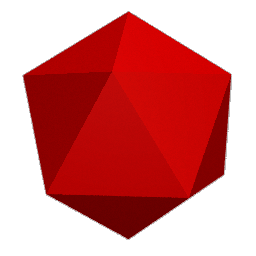# Making a icosahedron

Introduction -- Making the Modules -- Making the Polyhedra -- Jim's Origami Page
The icosahedron has 20 triangular faces that meet in groups of five at each of 12 vertices. It has 30 edges, and thus uses 30 identical triangle (tr--tr) modules.

You can color this like the dodecahedron, with ten modules each of three different colors. It can be colored so that all triangles have edges of each color.

Or you can color in a way analgous to the dodecahedron: All triangles meet in groups of five. Take five edges of color 1, and make one vertex of the icosahedron. This will make five incomplete triangles. Complete the triangles with color 2. Repeat this with the remaining five modules of color one, and the remaining five modules of color 2. Now you have made two pentagonal pyramids, which compose the top and the bottom of the icosahedron. Use color 3 for the remaining edges, which make a zig-zag around the middle.

## The icosahedronJim Plank --- Jim's Origami Page